# vectors (b)) Let a, b and c be three vectors such that a is perpendicular to both b and and Ibl = lel. Showr that th...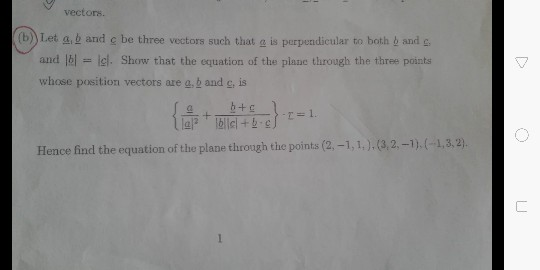vectors (b)) Let a, b and c be three vectors such that a is perpendicular to both b and and Ibl = lel. Showr that the equation of the plane through the three points whose position vectors are g, b and c, is le尸. }blicl+b.ef Hence find the equation of the plane through the points (2,-1,1,).(3,2,-1).(-1,3.2) ㄈ

As the name suggests, non collinear points refer to those points that do not all lie on the same line. From our knowledge from previous lessons, we know that an infinite number of planes can pass through a given vector that is perpendicular to it but there will always be one and only one plane that is perpendicular to the vector and passes through a given point.

In this lesson, we shall focus on the equation of a plane passing through three non collinear points. We shall look at both – the Vector form and the Cartesian form of equations.

Vector Form

Let us consider three non collinear points – R, S, T. Let the position vectors of these points be a⃗ ,bandc⃗ . We know that the product of the vectors RSandRT→ must be perpendicular to the plane containing the points R, S and T.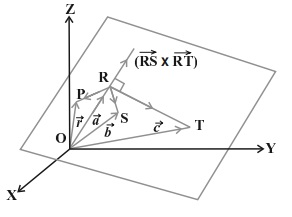Source: examfear

Let us also assume that the position vector of the point P is r⃗ and that of point R is a⃗ . Again, we know that the equation of the plane perpendicular to RS→×RT→ and passing through point P must be

(r⃗ –a⃗ ).(RS→×RT→)=0

From the figure we can write,

(r⃗ –a⃗ ).[(b⃗ –a⃗ )×(c⃗ –a⃗ )]=0

Note that here b⃗ and c⃗ are the position vectors of the points S and T respectively. Thus, the above equation can be taken to represent the equation of a plane passing through three non collinear points in Vector form.

We shall now move on to the Cartesian equation.

Cartesian Form

Let us now consider the coordinates of the three non collinear points as under –

(R(x1,y1,z1),S(x2,y2,z2),T(x3,y3,z3)

Let P be a point on the plane that must contain these points. The position vector of the point P be r⃗ and its coordinates are (x, y, z). Now, we can write the vectors as –

RP→=(xx1)i^+(yy1)j^+(zz1)k^

RS→=(x2–x1)i^+(y2–y1)j^+(z2–z1)k^

Also,

RP→=(x3–x1)i^+(y3–y1)j^+(z3–z1)k^

We can now use the Vector form to substitute the above vectors. Doing so would give us the advantage of presenting the equation in determinant form as under –

∣∣∣∣∣(xx1)(x2–x1)(x3–x1)(yy1)(y2–y1)(y3–y1)(zz1)(z2–z1)(z3–z1)∣∣∣∣∣=0

Thus the determinant form gives us nothing but the Cartesian equation of a plane passing through three non collinear points.

Solved Example for You

Question: Find the equation of the plane in Vector form that passes through the points (1, 1, 0), (1, 2, 1) and (-2, 2, -1).

Answer: We shall first check the determinant of the three points to check for collinearity of the points.

⎡⎣⎢11−212201−1⎤⎦⎥=−8

Since the value of the determinant is not zero, it implies that the points are non collinear. Now, we shall find the equation of the plane in Cartesian from as under –

∣∣∣∣∣(x–1)(1–1)(−2–1)(y–1)(2–1)(2–1)(z–0)(1–0)(−1–0)∣∣∣∣∣=0

∣∣∣∣(x–1)0−3(y–1)11z1−1∣∣∣∣=0

On solving, we shall get :

(x – 1) (-2) – (y – 1) (-3) + z (-3) = 0

-2x + 3y -3z -1 = 0

2x – 3y + 3z = -1 is the required equation of the plane.

##### Add Answer of: vectors (b)) Let a, b and c be three vectors such that a is perpendicular to both b and and Ibl = lel. Showr that th...
Similar Homework Help Questions
• ### Question 4 6-69) Consider the following three points: a-0 b-3and c-1 a) Find the parametric vector equation of the plane which passes through these three [3 marks] b) Find the vector cartesian equati...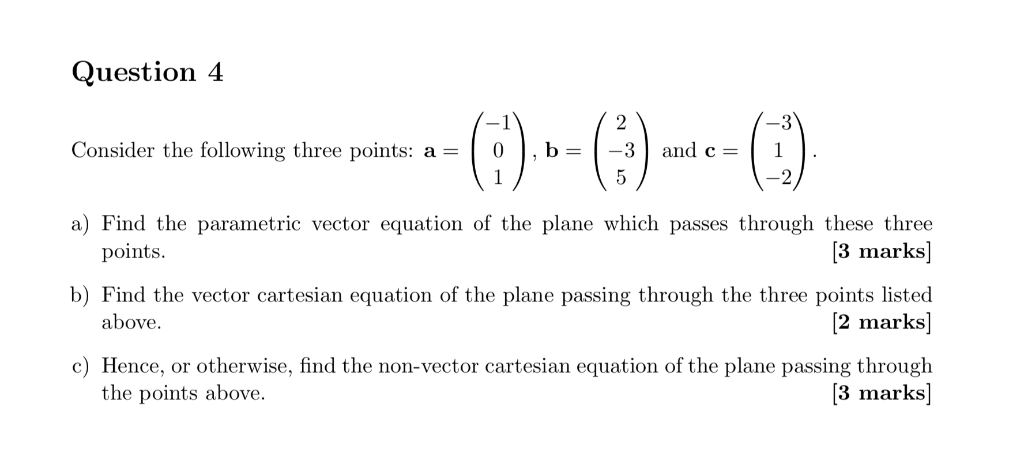Question 4 6-69) Consider the following three points: a-0 b-3and c-1 a) Find the parametric vector equation of the plane which passes through these three [3 marks] b) Find the vector cartesian equation of the plane passing through the three points listed [2 marks] c) Hence, or otherwise, find the non-vector cartesian equation of the plane passing through 3 marks] points above. the points above. Question 4 6-69) Consider the following three points: a-0 b-3and c-1 a) Find the parametric...

• ### prove that normal to plane containing 3 points whose position vectors are a vector,b vector,c vectorlies in direction addition of cross product of vectors b and c and cross product of vectors c and a and cross product of vectors a and b

prove that normal to plane containing 3 points whose position vectors are a vector,b vector,c vectorlies in direction addition of cross product of vectors b and c and cross product of vectors c and a and cross product of vectors a and b

• ### Consider the following vectors a-2i +j- 2k. b - 2i - 2j - 3k, Cー where i, j and k are Cartesian vectors. [7 (v) Fin...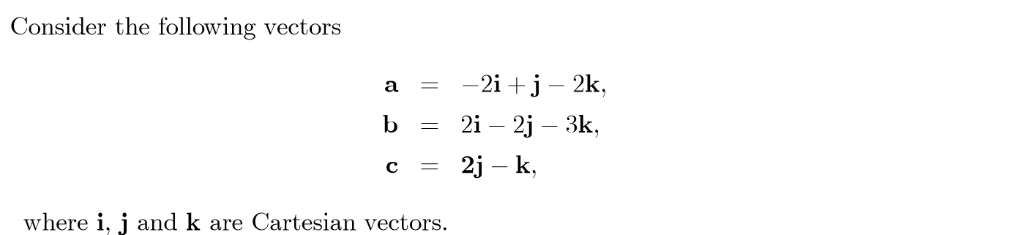Consider the following vectors a-2i +j- 2k. b - 2i - 2j - 3k, Cー where i, j and k are Cartesian vectors. [7 (v) Find the projections of vector c onto the directions of a, b and w vi) Show that the sum of these three projections obtained in (v) is equal to c.2 (b) A tetrahedron is a three-dimensional solid with a triangular base and three triangular faces. The formula of a pyramid applied also to a tetrahedron:...

• ### Problem l: Let u, v and w be three vectors in R3 (a) Prove that wlv +lvlw bisects the angle betwe...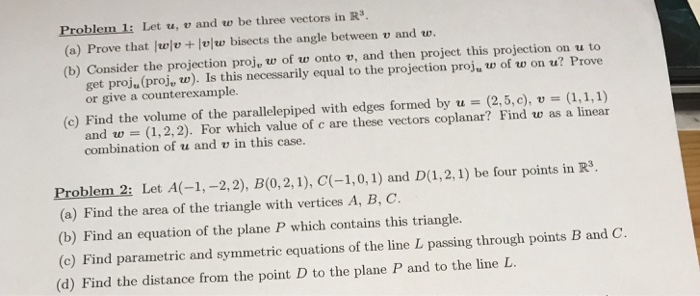Problem l: Let u, v and w be three vectors in R3 (a) Prove that wlv +lvlw bisects the angle between v and w. (b) Consider the projection proj, w of w onto v, and then project this projection on u to get proju (proj, w). Is this necessarily equal to the projection proj, w of w on u? Prove or give a counterexample. (c) Find the volume of the parallelepiped with edges formed by u-(2,5,c), v (1,1,1) and w...

• ### vectors

Let u = 2i - 3j + k and v = 4i + j - 2k(a) Find unit vectors in the direction of u and v;(b) Find an equation of the line through the point (2, 3, -4) in the direction of2u + 3v(c) Find dot product of vectors u and v;(d) Find an equation of the plane through the point (1, 0, 2) that contains bothvectors u and v.

• ### АЗ. You are given that the plane P contains both the point and the line Ls, where Q has position ...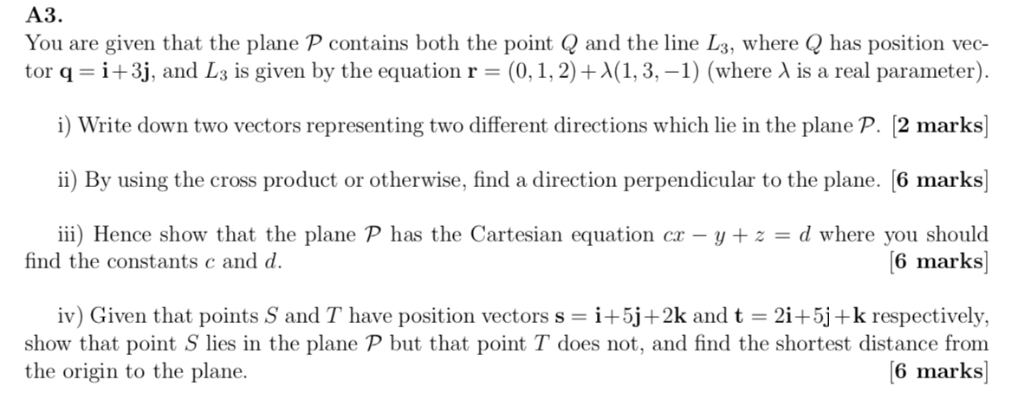АЗ. You are given that the plane P contains both the point and the line Ls, where Q has position vec- tor q = i + 3, and L3 is given by the equation r = (0, i, 2) + λ(1, 3,-1) (where λ is a real parameter). i) Write down two vectors representing two different directions which lie in the plane P. [2 marks i) By using the cross product or otherwise, find a direction perpendicular to the plane....

• ### unit vectors

Let u = 2i - 3j + k and v = 4i + j - 2k(a) Find unit vectors in the direction of u and v;(b) Find an equation of the line through the point (2; 3;-4) in the direction of2u + 3v;(c) Find dot product of vectors u and v;(d) Find an equation of the plane through the point (1; 0; 2) that contains bothvectors u and v.

• ### 2. Three charged particles, A, B, and C lie in a plane. Particle A has a...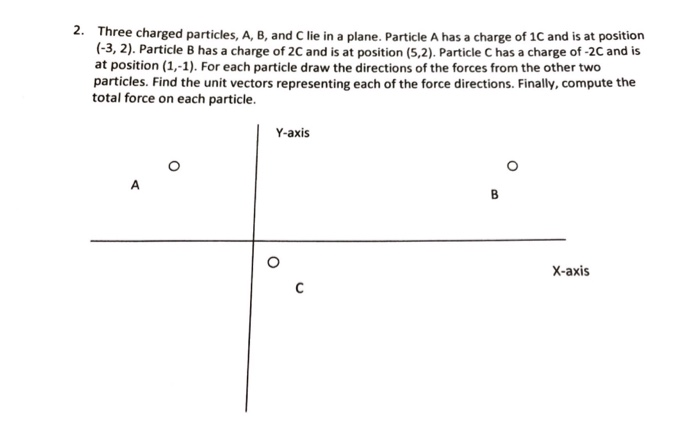2. Three charged particles, A, B, and C lie in a plane. Particle A has a charge of 1C and is at position -3,2). Particle B has a charge of 2C and is at position (5,2) Particle C has a charge of-2C and is at position (1,-1). For each particle draw the directions of the forces from the other two particles. Find the unit vectors representing each of the force directions. Finally, compute the total force on each particle. Y-axis...

• ### vi) The points A and B in R3 have position vectors a) Find a parametric vector...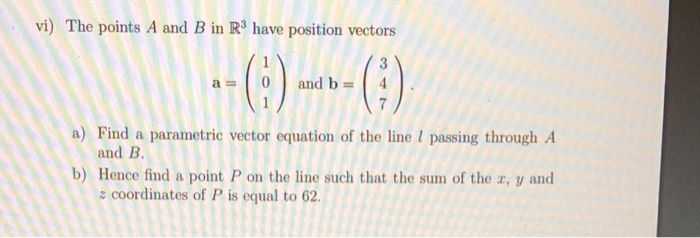vi) The points A and B in R3 have position vectors a) Find a parametric vector equation of the line I passing through A and B b) Hence find a point P on the line such that the sum of the z, y and ะ coordinates of P is equal to 62.

• ### An) a) Find the magnitude of both vectors. b) Find dot product and cross product of both vectors ...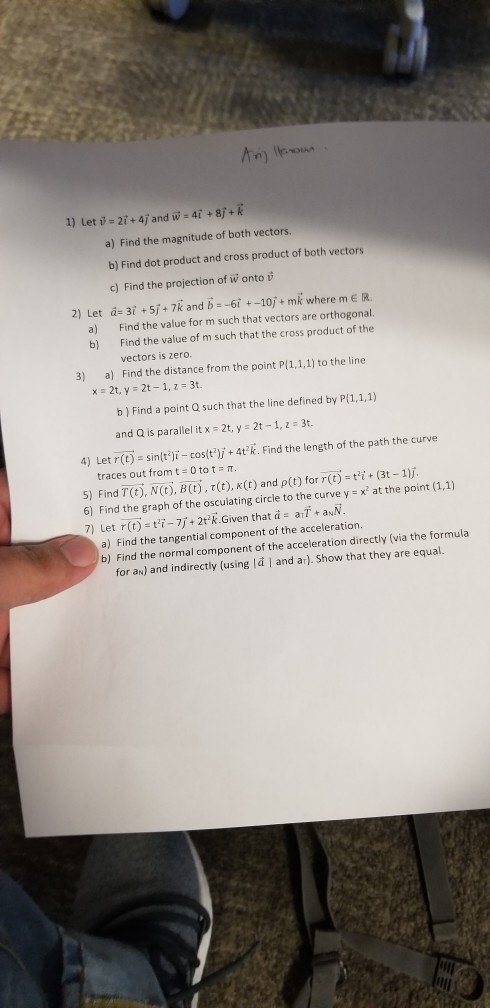pls answer 4,5,6 and 7 An) a) Find the magnitude of both vectors. b) Find dot product and cross product of both vectors c) Find the projection of w onto v 2) Let а:31 + 5, + 7k and b--6r +-10, + mk where m e R. a) Find the value for m such that vectors are orthogonal b) Find the value of m such that the cross product of the vectors is zero 3) a) Find the distance from...

Free Homework App